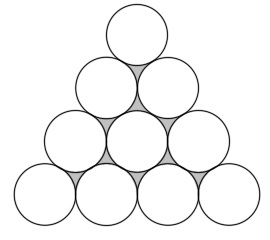# Ten circles

Geometry Level 2Ten circles of equal radii are arranged as shown. If the area of the shaded region is $9\sqrt{3}-\frac{9}{2}\pi$, what is the area of the ten circles?

×